# 一.高通濾波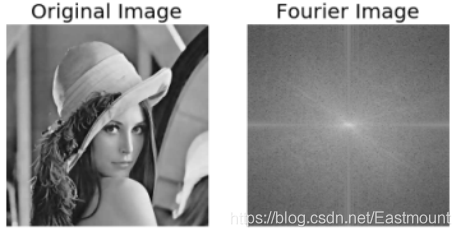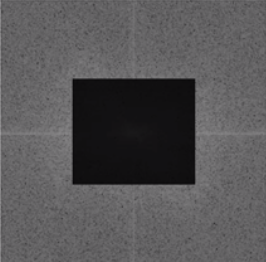``````rows, cols = img.shape
crow,ccol = int(rows/2), int(cols/2)
fshift[crow-30:crow+30, ccol-30:ccol+30] = 0
``````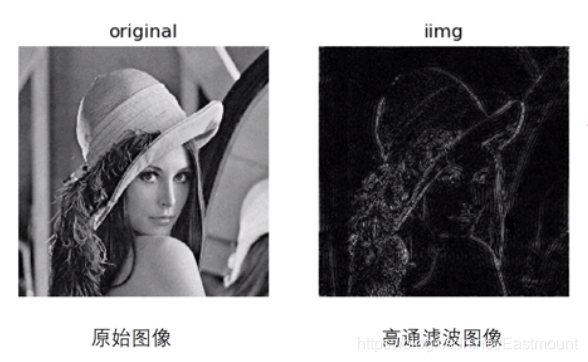``````# -*- coding: utf-8 -*-
import cv2 as cv
import numpy as np
from matplotlib import pyplot as plt

#讀取影象

#傅立葉變換
f = np.fft.fft2(img)
fshift = np.fft.fftshift(f)

#設定高通濾波器
rows, cols = img.shape
crow,ccol = int(rows/2), int(cols/2)
fshift[crow-30:crow+30, ccol-30:ccol+30] = 0

#傅立葉逆變換
ishift = np.fft.ifftshift(fshift)
iimg = np.fft.ifft2(ishift)
iimg = np.abs(iimg)

#顯示原始影象和高通濾波處理影象
plt.subplot(121), plt.imshow(img, 'gray'), plt.title('Original Image')
plt.axis('off')
plt.subplot(122), plt.imshow(iimg, 'gray'), plt.title('Result Image')
plt.axis('off')
plt.show()
``````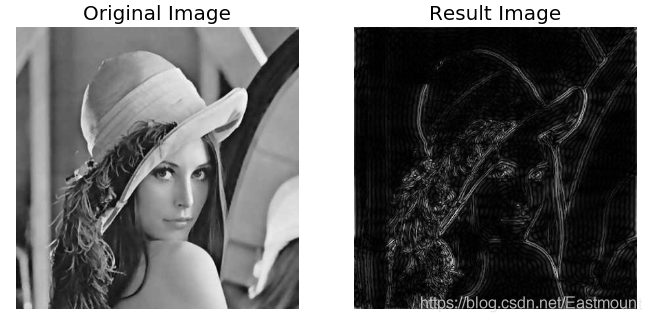# 二.低通濾波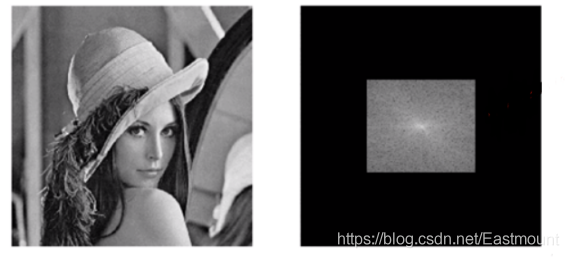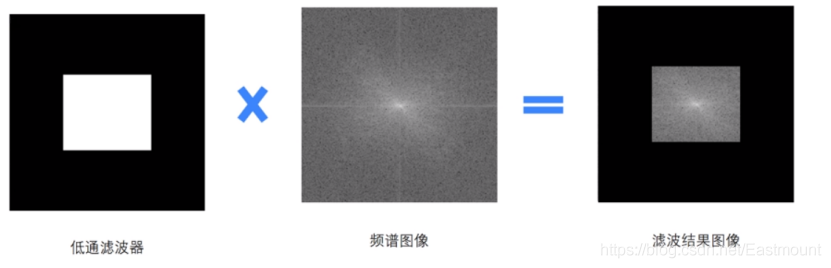``````rows, cols = img.shape
crow,ccol = int(rows/2), int(cols/2)
mask = np.zeros((rows, cols, 2), np.uint8)
``````

``````# -*- coding: utf-8 -*-
import cv2
import numpy as np
from matplotlib import pyplot as plt

#讀取影象

#傅立葉變換
dft = cv2.dft(np.float32(img), flags = cv2.DFT_COMPLEX_OUTPUT)
fshift = np.fft.fftshift(dft)

#設定低通濾波器
rows, cols = img.shape
crow,ccol = int(rows/2), int(cols/2) #中心位置
mask = np.zeros((rows, cols, 2), np.uint8)

#掩膜影象和頻譜影象乘積
#傅立葉逆變換
ishift = np.fft.ifftshift(f)
iimg = cv2.idft(ishift)
res = cv2.magnitude(iimg[:,:,0], iimg[:,:,1])

#顯示原始影象和低通濾波處理影象
plt.subplot(121), plt.imshow(img, 'gray'), plt.title('Original Image')
plt.axis('off')
plt.subplot(122), plt.imshow(res, 'gray'), plt.title('Result Image')
plt.axis('off')
plt.show()
``````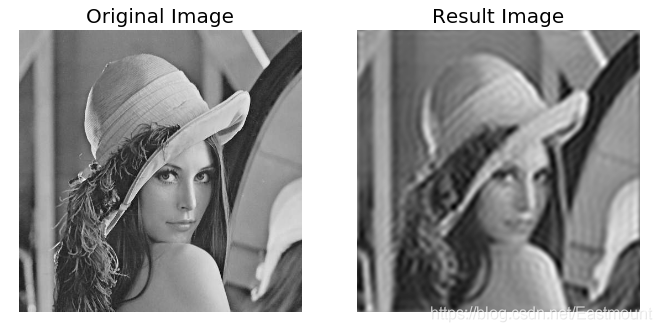• 《數字影象處理》（第3版），岡薩雷斯著，阮秋琦譯，電子工業出版社，2013年.
• 《數字影象處理學》（第3版），阮秋琦，電子工業出版社，2008年，北京.
• 《OpenCV3程式設計入門》，毛星雲，冷雪飛，電子工業出版社，2015，北京.
• 百度百科-傅立葉變換
• 網易雲課堂-高登教育 Python+OpenCV影象處理